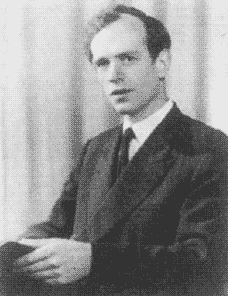Error-correcting codes can be used to construct interesting lattices, the best known example being the Leech lattice constructed from the binary Golay code. Recall that a lattice $L$ in $\mathbb{R}^n$ is the set of all integral linear combinations of n linearly independent vectors $\{ v_1,\ldots,v_n \}$, that is

$L = \mathbb{Z} v_1 \oplus \ldots \oplus \mathbb{Z} v_n$

The theta function of the lattice is the power series

$\Theta_L(q) = \sum_l a_l q^l$

with $a_l$ being the number of vectors in $L$ of squared length $l$. If all squared lengths are even integers, the lattice is called even and if it has one point per unit volume, we call it unimodular. The theta function of an even unimodular lattice is a modular form. One of the many gems from Conway’s book The sensual (quadratic) form is the chapter “Can You Hear the Shape of a Lattice?” or in other words, whether the theta function determines the lattice.Ernst Witt knew already that there are just two even unimodular lattices in 16 dimensions : $E_* \oplus E_8$ and $D_{16}^+$ and as there is just one modular form of weigth 8 upto scalars, the theta function cannot determine the latice in 16 dimensions. The number of dimensions for a counterexamle was sunsequently reduced to 12 (Kneser), 8 (Kitaoka),6 (Sloane) and finally 4 (Schiemann).

Sloane and Conway found an elegant counterexample in dimension 4 using two old friends : the tetracode and the taxicab number 1729 = 7 x 13 x 19.

Recall that the tetracode is a one-error correcting code consisting of the following nine words of length four over $\mathbb{F}_3 = \\{ 0,+,- \\}$

$\begin{matrix} 0~0 0 0 & 0~+ + + & 0~- – – \\\ +~0 + – & +~+ – 0 & +~- 0 + \\\ -~0 – + & -~+ 0 – & -~- + 0 \end{matrix}$

The first element (which is slightly offset from the rest) is the slope s of the words, and the other three digits cyclically increase by s (in the field $\mathbb{F}_3$). Now take four mutually orthogonal vectors in $\mathbb{R}^4$ with square lengths

$e_a.e_a = \frac{1}{12}, e_b.e_b = \frac{7}{12}, e_c.e_c = \frac{13}{12}, e_d.e_d= \frac{19}{12}$

and denote with $~(w,x,y,z)$ the vector $w e_a + x e_b + y e_c + z e_d$. Now consider the two lattices $L^+$ respectively $L^-$ spanned by the vectors

$~(3,-1,-1,-1),(1,3,1,-1),(1,-1,3,1),(1,1,-1,3)$ resp. $~(-3,-1,-1,-1),(1,-3,1,-1),(1,-1,-3,1),(1,1,-1,-3)$

then it follows that if we reduce any vector in either lattice modulo 3 we get a tetracode word. Using this fact it is not too difficult to show that there is a length preserving bijection between $L^+$ and $L^-$ given by the rule : change the sign of the first coordinate that is divisible by 3. As a direct consequence, the theta functions of these two lattices are equal.

Yet, these lattices cannot be isometric. One verfies that the only vectors of norm 4 in $L^+$ are $\pm (3,-1,-1,-1)$ and those of norm 8 are $\pm (1,3,1,-1)$ and one computes that their inproduct is

$~(3,-1,-1,-1).(1,3,1,-1)=-1$

Similarly, the only vectors of norm 4 in $L^-$ are $\pm (-3,-1,-1,-1)$ and those of norm 8 are $\pm (1,-3,1,-1)$ whereas their inproduct is

$~(-3,-1,-1,-1).(1,-3,1,-1) = 2$

so the two lattices are different!

Reference

John H. Conway, “The sensual (quadratic) form” second lecture “Can you hear the shape of a lattice?”

Published in featured